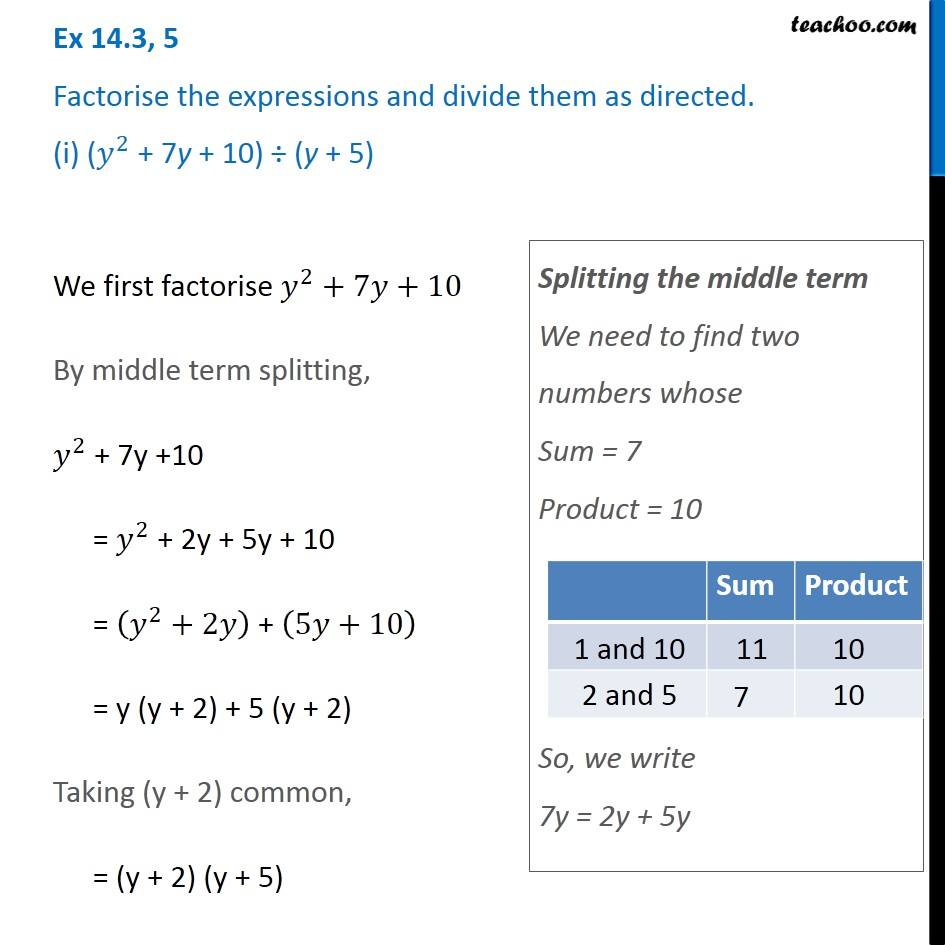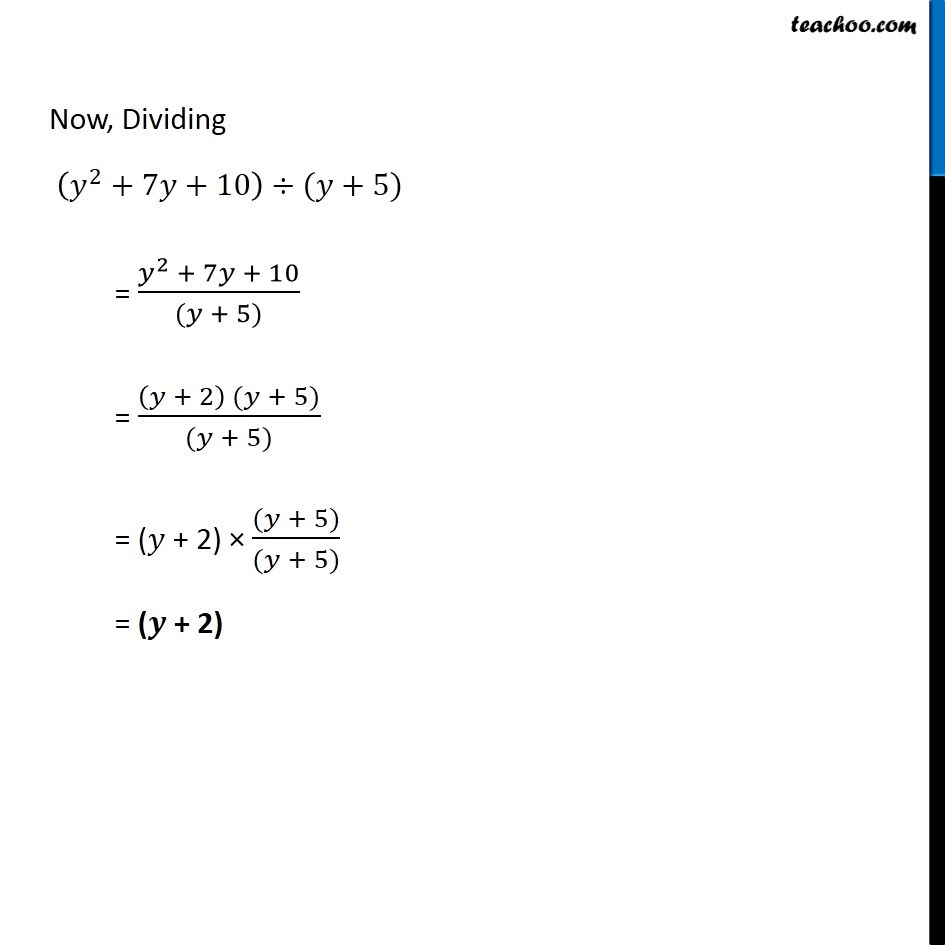Dividing two polynomials

Chapter 14 Class 8 Factorisation
Concept wiseIntroducing your new favourite teacher - Teachoo Black, at only ₹83 per month

### Transcript

Ex 14.3, 5 Factorise the expressions and divide them as directed. (i) (𝑦^2 + 7y + 10) ÷ (y + 5) We first factorise 𝑦^2+7𝑦+10 By middle term splitting, 𝑦^2 + 7y +10 = 𝑦^2 + 2y + 5y + 10 = (𝑦^2+2𝑦) + (5𝑦+10) = y (y + 2) + 5 (y + 2) Taking (y + 2) common, = (y + 2) (y + 5) Splitting the middle term We need to find two numbers whose Sum = 7 Product = 10 So, we write 7y = 2y + 5y Now, Dividing (𝑦^2+7𝑦+10)÷(𝑦+5) = (𝑦^2 + 7𝑦 + 10)/((𝑦 + 5)) = ((𝑦 + 2) (𝑦 + 5))/((𝑦 + 5)) = (𝑦 + 2) × ((𝑦 + 5))/((𝑦 + 5)) = (𝒚 + 2)